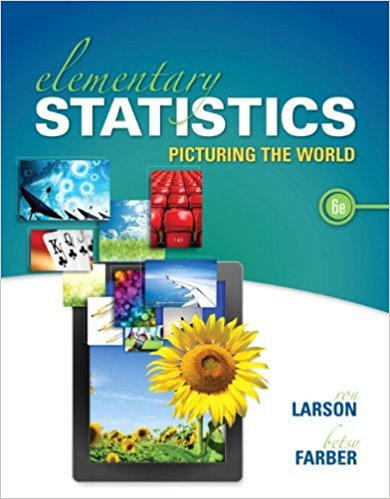×
×

# Solutions for Chapter 3: PROBABILITY## Full solutions for Elementary Statistics: Picturing the World | 6th Edition

ISBN: 9780321911216Solutions for Chapter 3: PROBABILITY

Solutions for Chapter 3
4 5 0 382 Reviews
13
1
##### ISBN: 9780321911216

This expansive textbook survival guide covers the following chapters and their solutions. This textbook survival guide was created for the textbook: Elementary Statistics: Picturing the World , edition: 6. Elementary Statistics: Picturing the World was written by and is associated to the ISBN: 9780321911216. Since 53 problems in chapter 3: PROBABILITY have been answered, more than 100947 students have viewed full step-by-step solutions from this chapter. Chapter 3: PROBABILITY includes 53 full step-by-step solutions.

Key Statistics Terms and definitions covered in this textbook
• Analytic study

A study in which a sample from a population is used to make inference to a future population. Stability needs to be assumed. See Enumerative study

• C chart

An attribute control chart that plots the total number of defects per unit in a subgroup. Similar to a defects-per-unit or U chart.

• Causal variable

When y fx = ( ) and y is considered to be caused by x, x is sometimes called a causal variable

• Combination.

A subset selected without replacement from a set used to determine the number of outcomes in events and sample spaces.

• Conditional probability

The probability of an event given that the random experiment produces an outcome in another event.

• Conditional probability density function

The probability density function of the conditional probability distribution of a continuous random variable.

• Conditional variance.

The variance of the conditional probability distribution of a random variable.

• Conidence interval

If it is possible to write a probability statement of the form PL U ( ) ? ? ? ? = ?1 where L and U are functions of only the sample data and ? is a parameter, then the interval between L and U is called a conidence interval (or a 100 1( )% ? ? conidence interval). The interpretation is that a statement that the parameter ? lies in this interval will be true 100 1( )% ? ? of the times that such a statement is made

• Continuity correction.

A correction factor used to improve the approximation to binomial probabilities from a normal distribution.

• Crossed factors

Another name for factors that are arranged in a factorial experiment.

• Defect concentration diagram

A quality tool that graphically shows the location of defects on a part or in a process.

• Dependent variable

The response variable in regression or a designed experiment.

• Design matrix

A matrix that provides the tests that are to be conducted in an experiment.

• Erlang random variable

A continuous random variable that is the sum of a ixed number of independent, exponential random variables.

• Error propagation

An analysis of how the variance of the random variable that represents that output of a system depends on the variances of the inputs. A formula exists when the output is a linear function of the inputs and the formula is simpliied if the inputs are assumed to be independent.

• Estimate (or point estimate)

The numerical value of a point estimator.

• Exponential random variable

A series of tests in which changes are made to the system under study

• Extra sum of squares method

A method used in regression analysis to conduct a hypothesis test for the additional contribution of one or more variables to a model.

• First-order model

A model that contains only irstorder terms. For example, the irst-order response surface model in two variables is y xx = + ?? ? ? 0 11 2 2 + + . A irst-order model is also called a main effects model

• Geometric random variable

A discrete random variable that is the number of Bernoulli trials until a success occurs.

×